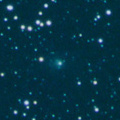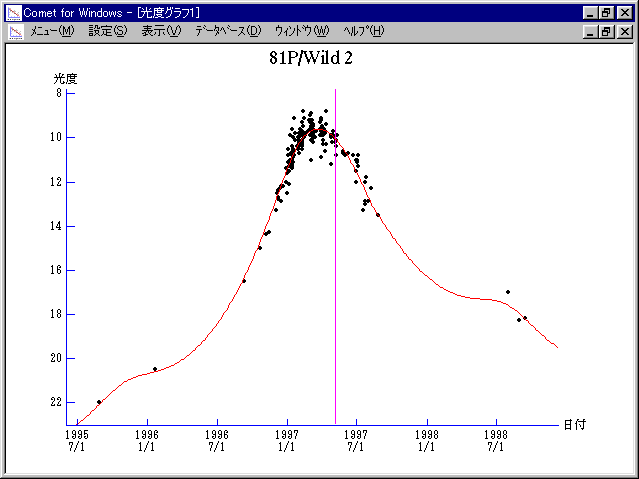\$B%t%#%k%HBh#2WB@1(B

81P/Wild 2 (1997)\$B\$B50F;MWAG(B

```   The following improved orbital elements by Kenji Muraoka,
are from 760 observations 1978 to 1997, including 9 Planets,
Moon and 5 minor planets perturbations and non-gravitational
effect of style II.  The mean residual is +/- 0.80 arc seconds.

Epoch  =  1997 Apr. 22.0  TT       JDT = 2450560.5
T  =  1997 May   6.62722       +/- 0.00017 (m.e.) TT
Peri. =   41.76780                +/- 0.00013
Node  =  136.15645                +/- 0.00010   (2000.0)
Incl. =    3.24261                +/- 0.00001
q  =    1.5826222              +/- 0.0000006 AU
e  =    0.5402209              +/- 0.0000002
a  =    3.4421362              +/- 0.0000006 AU
n  =    0.15433414             +/- 0.00000004
P  =    6.386                  +/- 0.0000017  years
A1  =   +0.113                  +/- 0.006
A2  =   +0.02089                +/- 0.00023
```\$B@1?^(B1996\$BG/(B 1\$B7n(B 8\$BF|!A(B1997\$BG/(B 6\$B7n(B11\$BF|(B1997\$BG/(B 6\$B7n(B11\$BF|!A(B1998\$BG/(B 4\$B7n(B27\$BF|(B\$B8wEYJQ2=(B

```        m1 = 5.3 + 5 log\$B&\$(B + 21.6 log r(t + 20)  [  ,95]  (              \$B!A(B1997\$BG/(B 8\$B7n(B 9\$BF|(B)
m1 = 8.2 + 5 log\$B&\$(B + 12.5 log r          [95,  ]  (1997\$BG/(B 8\$B7n(B 9\$BF|!A(B              )
```\$B50F;MWAG\$OB<2,7r<#;a\$N7W;;\$K\$h\$k\$b\$N\$G\$9!#(B \$B@1?^\$O(B StellaNavigator Ver.2.0 for Windows (\$B%"%9%H%m%"!<%D(B \$BJTCx(B / \$B%"%9%-!<=PHG6I4)(B) \$B\$G:n@.\$7\$?\$b\$N\$G\$9!#(B \$B8wEY%0%i%U\$O(BComet for Windows\$B\$G:n@.\$7\$?\$b\$N\$G\$9!#(B